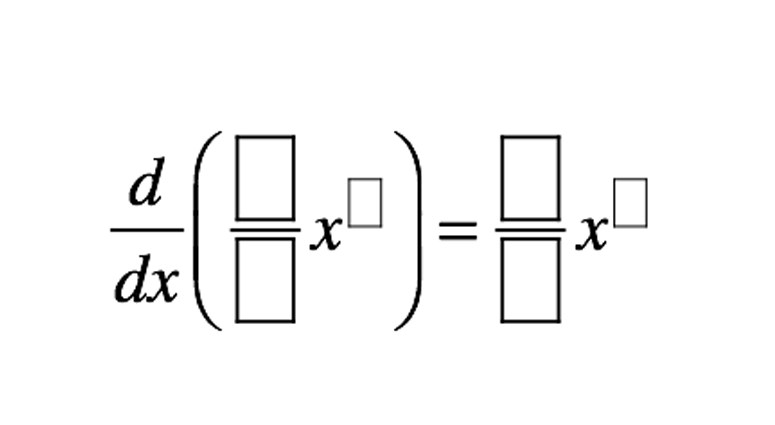Home > Calculus > Derivative Power Rule

# Derivative Power Rule

Directions: Use the digits 1 to 9, at most one time each, to fill in the boxes to create a true derivative statement.### Hint

What is true about the relationship between a function’s degree and its derivative?

What values in the coefficient could work with the exponent to not reuse any numbers?

There are multiple answers (at least 12) to the problem. Below are a few examples.

1. d/dx(1/8)x^6 = (3/4)x^5
2. d/dx(2/3)x^9= (6/1)x^8
3. d/dx(1/6)x^8=(4/3)x^7

Extension: What numbers would not work as exponents?

Source: Melissa Flynn

## Derivative of Trig Functions 2

Directions: Fill in the boxes below using the digits 1 to 6, at most one …